## What is impedance?

Before we get the speaker impedance curve explained, let’s get down to the basics. When you apply a voltage, an electrical system will exhibit a certain amount of opposition to current. We call this opposition impedance, and it is measured in ohms. Let’s make a distinction between impedance and resistance, which both share the same unit of measurement (ohms), but are not the same thing.

• Resistance is used when the circuit is driven by DC current. It will have a fixed value.
• Impedance is used when the circuit is driven by AC current. Because the current is alternating, the impedance will have not only magnitude, but phase also.

A speaker system uses AC current, so impedance is our culprit. Impedance changes value with frequency. When you take a look at a speaker’s spreadsheet, you might see both values of resistance and impedance. You might see something like Re=3.4 ohms (resistance) and Z=4 ohms (impedance). Re is the DC resistance of the voice coil and Z is the nominal impedance. The term nominal signifies the mean value, because the impedance changes with frequency. This means that the impedance will mostly be around 4 ohms, but it can reach values as high as 50 ohms are go as low as 2.5 ohms.

### Speaker impedance curve explained

Speaker impedance is not a single value, instead, it changes with frequency. This means that you will need a graph to accurately tell the impedance of a driver. Also, since the enclosure will affect the properties of the speaker, the impedance graph will change also. Let’s get the speaker impedance curve explained for a driver in free air and for a driver in a sealed enclosure.

### Driver in free air and sealed enclosure

Let’s analyze the impedance chart of a driver in a sealed enclosure. If the driver is in free air or in a closed box, the impedance chart will look similar. The spike in impedance will be at a higher frequency, because the enclosure will raise the resonant frequency of the system.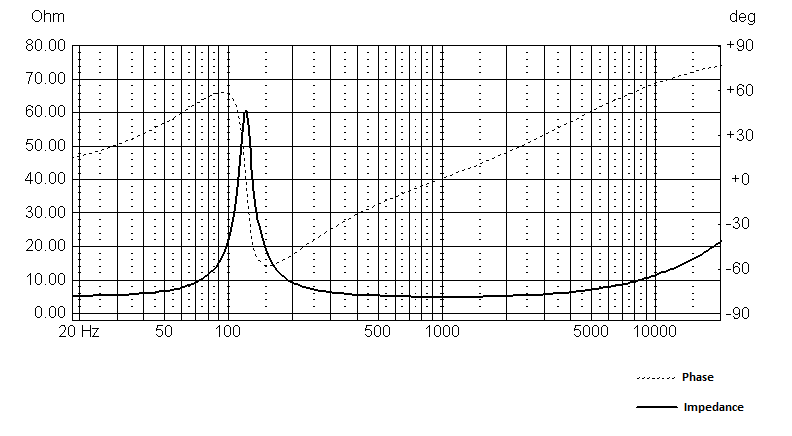• The bold line is the impedance. We measure the magnitude of the impedance in ohms, and you can read the value on the left side of the graph.
• The dotted line is the phase. We measure phase angle in degrees, and you can read the value on the right side of the graph.

### Impedance

There are several ques in the graph that reveal useful information, so let’s get the speaker impedance curve explained :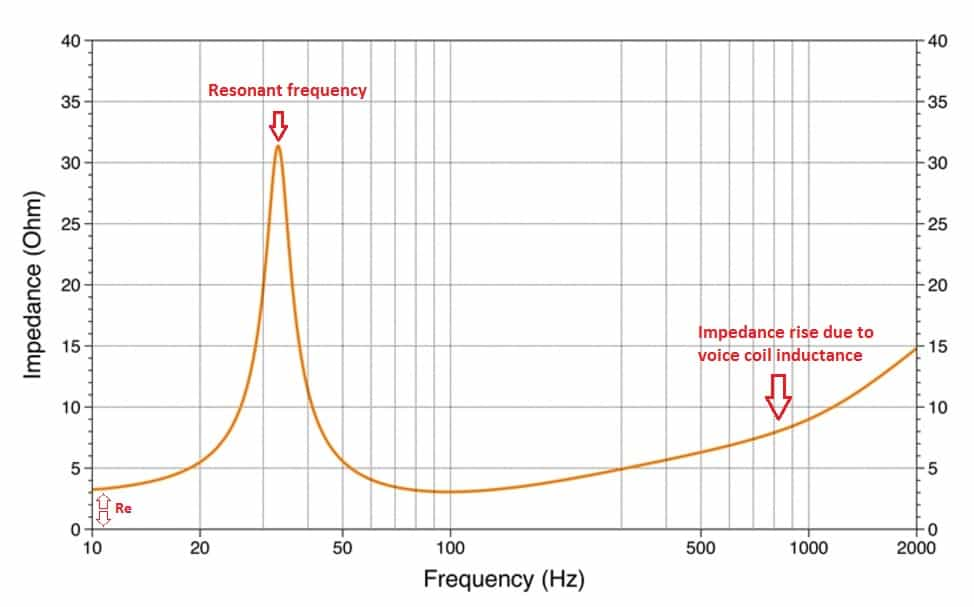• The lowest value on the left of the chart is the DC resistance (Re). Which in our graph looks like 3.2 ohms.
• The manufacturer quotes the nominal impedance, but that is the mean value of the impedance. Just a few ticks higher than Re. In our case, the nominal impedance is 4 ohms.
• The peak in the impedance chart represents the resonant frequency. Either for a driver in free-air or for a driver in a sealed enclosure, the peak will tell the resonant frequency of the system. For the closed enclosure the resonant frequency will be slightly higher, if we compare it to the same driver in free air.
• As the frequency goes up, so does the impedance. The speaker has a voice coil in its construction, which is an inductor. This will exhibit an inductive reactance, which opposes the current flow. Since this inductive reactance is directly proportional to frequency, this explains the impedance rise with frequency.

### Phase angle

In a reactive circuit (which has inductive and capacitive properties), there will sometimes be a phase mismatch between current and voltage. This means that the current will lead or lag the voltage by a certain time period. This difference is called phase angle and it’s measured in degrees.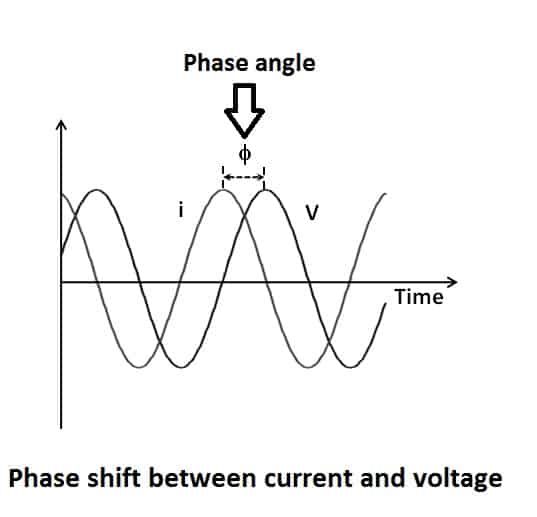Phase angle characteristics :

• In a capacitive circuit, the current will lead the voltage, resulting in a negative phase angle.
• The current will lag behind the voltage, in an inductive circuit, resulting in a positive phase angle.
• In a purely resistive circuit, the current and voltage are in phase, resulting in a 0 phase angle.

Now let’s get back to the audio world and let’s get the speaker impedance curve explained :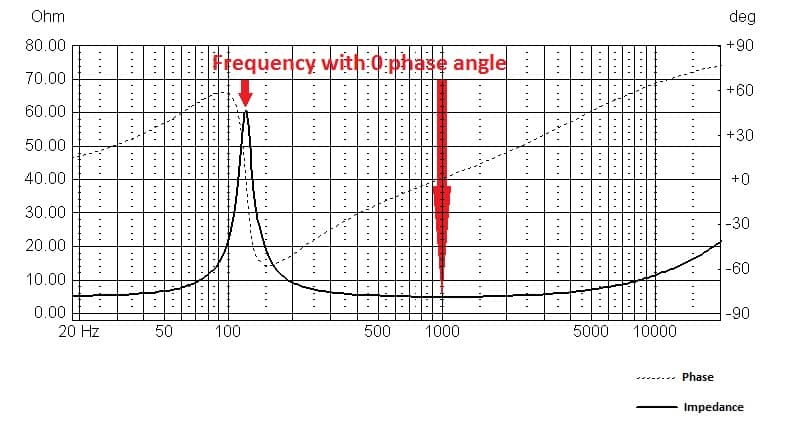Depending on how reactive the speaker system is, the phase angle will take different values. The phase angle will have 0 degrees at the resonant frequency and at the point where the impedance starts to rise due to voice coil inductance.

### How to design loudspeakers - video courses

What does this phase angle do in the real world? Well, it actually tells us how much heat the amplifier will dissipate in correlation to how much power it will deliver to a certain load (the speaker). The phase angle will vary from -90 degrees to +90 degrees, but in the real world it will have a narrower range : [-45 ; +45]. At 0 degrees  the amplifier will deliver as much power as it dissipates as heat. In the worst case scenario, 45 degree angle, the amplifier will heat up 4 times as much as the power it delivers. So if the amplifier delivers 100 W of power to the speaker (at a 45 degree phase shift), it will heat up like it’s delivering 400 W.

### Bass reflex impedance charts

Unlike with free-air and sealed enclosure impedance charts, the bass reflex impedance graph will show 2 peaks . Here is an example :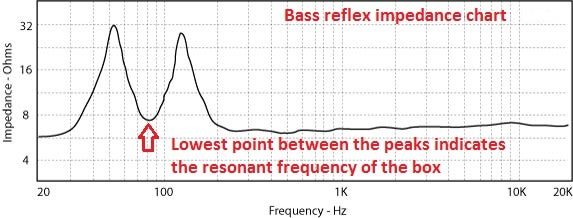The point between the peaks which has the lowest impedance, marks the resonant frequency of the box.

Depending on how the 2 peaks compare to each other, in terms of height, we can consider 3 particular cases :

1. The two peaks match in height. This means that the resonant frequency of the driver in free air (fs) matches the resonant frequency of the box (fb).
2. The first peak is higher than the second. This means that fb > fs.
3. The second peak is higher than the first peak. This means that fb < fs.

### Conclusion

Now that we got the speaker impedance curve explained, you can read in between the lines when you see one. Whether it’s the driver in free-air, acoustic suspension enclosure or bass reflex box, the impedance chart tells all kinds of useful information, if you know where to look for them.

#### References

1. The Complete Guide to High-End Audio 5th Edition by Robert Harley (Acapella Publishing, 2015).
2. Audio Engineering Explained by Douglas Self (Taylor & Francis, 2012).